# Linear Regression in R

This article explains how to run linear regression in R. This tutorial covers assumptions of linear regression and how to treat if assumptions violate. It also covers fitting the model and calculating model performance metrics to check the performance of linear regression model. Linear Regression is one of the most popular statistical technique. It has been in use for more than 3 decades. It is widely accepted in almost every domain as it's easy to understand output of linear regression.

Linear Regression
It is a way of finding a relationship between a single, continuous variable called Dependent or Target variable and one or more other variables (continuous or not) called Independent Variables.Linear Regression
It's a straight line curve. In the above figure, diagonal red line is a regression line which is also called best-fitting straight line. The distance between dots and regression line is errors. Linear regression aims at finding best fitting straight line by minimizing the sum of squared vertical distance between dots and regression line.

Variable Type
Linear regression requires the dependent variable to be continuous i.e. numeric values (no categories or groups).
Simple vs. Multiple Linear Regression
Linear regression can be simple linear regression when you have only one independent variable . Whereas Multiple linear regression will have more than one independent variable.
Regression Equation
Interpretation:
b0 is the intercept the expected mean value of dependent variable (Y) when all independent variables (Xs) are equal to 0. and b1 is the slope.
b1 represents the amount by which dependent variable (Y) changes if we change X1 by one unit keeping other variables constant.

Important Term : Residual
The difference between an observed (actual) value of the dependent variable and the value of the dependent variable predicted from the regression line.

Algorithm
Linear regression is based on least square estimation which says regression coefficients (estimates) should be chosen in such a way that it minimizes the sum of the squared distances of each observed response to its fitted value.
Minimum Sample Size
Linear regression requires 5 cases per independent variable in the analysis.
Assumptions of Linear Regression Analysis

1. Linear Relationship : Linear regression needs a linear relationship between the dependent and independent variables.

2. Normality of Residual : Linear regression requires residuals should be normally distributed.

3. Homoscedasticity :  Linear regression assumes that residuals are approximately equal for all predicted dependent variable values. In other words, it means constant variance of errors.

4. No Outlier Problem

5. Multicollinearity : It means there is a high correlation between independent variables. The linear regression model MUST NOT be faced with problem of multicollinearity.

6. Independence of error terms - No Autocorrelation

It states that the errors associated with one observation are not correlated with the errors of any other observation. It is a problem when you use time series data. Suppose you have collected data from labors in eight different districts. It is likely that the labors within each district will tend to be more like one another that labors from different districts, that is, their errors are not independent.

If you want to know how to check these assumptions and how to treat them if violated, check out this tutorial -  Checking the assumptions and Treatment to Violations of Assumptions

Distribution of Linear Regression
Linear regression assumes target or dependent variable to be normally distributed. Normal Distribution is same as Gaussian distribution. It uses identity link function of gaussian family

Standardized Coefficients

The concept of standardization or standardized coefficients (aka estimates) comes into picture when predictors (aka independent variables) are expressed in different units. Suppose you have 3 independent variables - age, height and weight. The variable 'age' is expressed in years, height in cm, weight in kg. If we need to rank these predictors based on the unstandardized coefficient, it would not be a fair comparison as the unit of these variable is not same.
Standardized Coefficients (or Estimates) are mainly used to rank predictors (or independent or explanatory variables) as it eliminate the units of measurement of  independent and dependent variables). We can rank independent variables with absolute value of standardized coefficients. The most important variable will have maximum absolute value of standardized coefficient.Standardized Coefficient for Linear Regression Model

Interpretation of Standardized Coefficient
A standardized coefficient value of 1.25 indicates that a change of one standard deviation in the independent variable results in a 1.25 standard deviations increase in the dependent variable.

Measures of Model Performance

1. R-squared
It measures the proportion of the variation in your dependent variable explained by all of your independent variables in the model. It assumes that every independent variable in the model helps to explain variation in the dependent variable. In reality, some variables don't affect dependent variable and they don't help building a good model.R-Squared Formula
In the numerator of equation above, yi-hat is the predicted value. Mean value of Y appears in denominator.

Rule :
Higher the R-squared, the better the model fits your data. In psychological surveys or studies, we generally found low R-squared values lower than 0.5. It is because we are trying to predict human behavior and it is not easy to predict humans. In these cases, if your R-squared value is low but you have statistically significant independent variables (aka predictors), you can still generate insights about how changes in the predictor values are associated with changes in the response value.
Can R-Squared be negative?

Yes, it is when horizontal line explains the data better than your model. It mostly happens when you do not include intercept. Without an intercept, the regression could do worse than the sample mean in terms of predicting the target variable. It is not only because of exclusion of intercept. It can be negative even with inclusion of intercept.

Mathematically, it is possible when error sum-of-squares from the model is larger than the total sum-of-squares from the horizontal line.
R-squared = 1 - [(Sum of Square Error)/(Total Sum of Square)]

It measures the proportion of variation explained by only those independent variables that really affect the dependent variable. It penalizes you for adding independent variable that do not affect the dependent variable.Adjusted R-Squared

Adjusted R-Squared is more important metrics than R-squared
Every time you add a independent variable to a model, the R-squared increases, even if the independent variable is insignificant. It never declines. Whereas Adjusted R-squared increases only when independent variable is significant and affects dependent variable.
3. RMSE ( Root Mean Square Error)

It explains how close the actual data points are to the model’s predicted values. It measures standard deviation of the residuals.RMSE Calculation
In the formula above, yi is the actual values of dependent variable and yi-hat is the predicted values, n - sample size.

Important Point
RMSE has the same unit as dependent variable. For example, dependent variable is sales which is measured in dollars. Let's say RMSE of this sales model comes out 21. We can say it is 21 dollars.

What is good RMSE score?

There is no thumb rule regarding good or bad RMSE score. It is because it is dependent on your dependent variable. If your target variable lies between 0 to 100. RMSE of 0.5 can be considered as good but same 0.5 RMSE can be considered as a poor score if dependent variable ranges from 0 to 10. Hence there is so such good or bad RMSE by simply looking at the value.

Lower values of RMSE indicate better fit. RMSE is a good measure of how accurately the model predicts the response, and is the most important criterion for fit if the main purpose of the model is prediction.
The RMSE for your training and your test sets should be very similar if you have built a good model. If the RMSE for the test set is much higher than that of the training set, it is likely that you've badly over fit the data, i.e. you've created a model that works well in sample, but has little predictive value when tested out of sample

RMSE vs MAE

RMSE amplifies and severely punishes large errors as compared to mean absolute error (MAE).

R-Squared vs RMSE
R-squared is in proportion and has no units associated to target variable whereas RMSE has units associated to target variable. Hence, R-squared is a relative measure of fit, RMSE is an absolute measure of fit.
The code below covers the assumption testing and evaluation of model performance :
1. Data Preparation
2. Testing of Multicollinearity
3. Treatment of Multicollinearity
4. Checking for Autocorrelation
5. Checking for Outliers
6. Checking for Heteroscedasticity
7. Testing of Normality of Residuals
8. Forward, Backward and Stepwise Selection
9. Calculating RMSE
10. Box Cox Transformation of Dependent Variable
11. Calculating R-Squared and Adj, R-squared manually
12. Calculating Residual and Predicted values
13. Calculating Standardized Coefficient

R Script : Linear Regression

Theory part is over. Let's implement linear regression with  R -

library(ggplot2)
library(car)
library(caret)
library(corrplot)
Make sure the above listed packages are already installed and loaded into R. If they are not already installed, you need to install it by using the command install.packages("package-name")

We will use mtcars dataset from cars package. This data was extracted from the Motor Trend US magazine, and comprises fuel consumption and 10 aspects of automobile design and performance for 32 automobiles.
data(mtcars)

# Looking at variables
str(mtcars)
```'data.frame': 32 obs. of  11 variables:
\$ mpg : num  21 21 22.8 21.4 18.7 18.1 14.3 24.4 22.8 19.2 ...
\$ cyl : num  6 6 4 6 8 6 8 4 4 6 ...
\$ disp: num  160 160 108 258 360 ...
\$ hp  : num  110 110 93 110 175 105 245 62 95 123 ...
\$ drat: num  3.9 3.9 3.85 3.08 3.15 2.76 3.21 3.69 3.92 3.92 ...
\$ wt  : num  2.62 2.88 2.32 3.21 3.44 ...
\$ qsec: num  16.5 17 18.6 19.4 17 ...
\$ vs  : num  0 0 1 1 0 1 0 1 1 1 ...
\$ am  : num  1 1 1 0 0 0 0 0 0 0 ...
\$ gear: num  4 4 4 3 3 3 3 4 4 4 ...
\$ carb: num  4 4 1 1 2 1 4 2 2 4 ...
```
Variable description of the above variables are listed below against their respective variable names.

Variable Description
mpg Miles/(US) gallon
cyl Number of cylinders
disp Displacement (cu.in.)
hp Gross horsepower
drat Rear axle ratio
wt Weight (1000 lbs)
qsec 1/4 mile time
vs V/S
am Transmission (0 = automatic, 1 = manual)
gear Number of forward gears
carb Number of carburetors

Summarize Data

In this dataset, mpg is a target variable. See first 6 rows of data by using head() function.

```> head(mtcars)
mpg cyl disp  hp drat    wt  qsec vs am gear carb
Mazda RX4         21.0   6  160 110 3.90 2.620 16.46  0  1    4    4
Mazda RX4 Wag     21.0   6  160 110 3.90 2.875 17.02  0  1    4    4
Datsun 710        22.8   4  108  93 3.85 2.320 18.61  1  1    4    1
Hornet 4 Drive    21.4   6  258 110 3.08 3.215 19.44  1  0    3    1
Hornet Sportabout 18.7   8  360 175 3.15 3.440 17.02  0  0    3    2
Valiant           18.1   6  225 105 2.76 3.460 20.22  1  0    3    1```

To see the distribution of the variables, submit summary() function.
summary(mtcars)
```> summary(mtcars)
mpg             cyl             disp             hp
Min.   :10.40   Min.   :4.000   Min.   : 71.1   Min.   : 52.0
1st Qu.:15.43   1st Qu.:4.000   1st Qu.:120.8   1st Qu.: 96.5
Median :19.20   Median :6.000   Median :196.3   Median :123.0
Mean   :20.09   Mean   :6.188   Mean   :230.7   Mean   :146.7
3rd Qu.:22.80   3rd Qu.:8.000   3rd Qu.:326.0   3rd Qu.:180.0
Max.   :33.90   Max.   :8.000   Max.   :472.0   Max.   :335.0
drat             wt             qsec             vs
Min.   :2.760   Min.   :1.513   Min.   :14.50   Min.   :0.0000
1st Qu.:3.080   1st Qu.:2.581   1st Qu.:16.89   1st Qu.:0.0000
Median :3.695   Median :3.325   Median :17.71   Median :0.0000
Mean   :3.597   Mean   :3.217   Mean   :17.85   Mean   :0.4375
3rd Qu.:3.920   3rd Qu.:3.610   3rd Qu.:18.90   3rd Qu.:1.0000
Max.   :4.930   Max.   :5.424   Max.   :22.90   Max.   :1.0000
am              gear            carb
Min.   :0.0000   Min.   :3.000   Min.   :1.000
1st Qu.:0.0000   1st Qu.:3.000   1st Qu.:2.000
Median :0.0000   Median :4.000   Median :2.000
Mean   :0.4062   Mean   :3.688   Mean   :2.812
3rd Qu.:1.0000   3rd Qu.:4.000   3rd Qu.:4.000
Max.   :1.0000   Max.   :5.000   Max.   :8.000
```

Data Preparation

Make sure categorical variables are stored as factors. In the program below, we are converting variables to factors.
mtcars\$am   = as.factor(mtcars\$am)
mtcars\$cyl  = as.factor(mtcars\$cyl)
mtcars\$vs   = as.factor(mtcars\$vs)
mtcars\$gear = as.factor(mtcars\$gear)
Identifying and Correcting Collinearity

In this step, we are identifying independent variables which are highly correlated to each other. Since mpg is a dependent variable, we are removing it in the code below.
```#Dropping dependent variable for calculating Multicollinearity
mtcars_a = subset(mtcars, select = -c(mpg))

#Identifying numeric variables
numericData <- mtcars_a[sapply(mtcars_a, is.numeric)]

#Calculating Correlation
descrCor <- cor(numericData)

# Print correlation matrix and look at max correlation
print(descrCor)
disp         hp        drat         wt        qsec       carb
disp  1.0000000  0.7909486 -0.71021393  0.8879799 -0.43369788  0.3949769
hp    0.7909486  1.0000000 -0.44875912  0.6587479 -0.70822339  0.7498125
drat -0.7102139 -0.4487591  1.00000000 -0.7124406  0.09120476 -0.0907898
wt    0.8879799  0.6587479 -0.71244065  1.0000000 -0.17471588  0.4276059
qsec -0.4336979 -0.7082234  0.09120476 -0.1747159  1.00000000 -0.6562492
carb  0.3949769  0.7498125 -0.09078980  0.4276059 -0.65624923  1.0000000

# Visualize Correlation Matrix
corrplot(descrCor, order = "FPC", method = "color", type = "lower", tl.cex = 0.7, tl.col = rgb(0, 0, 0))
```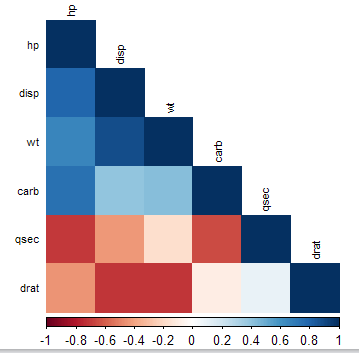Correlation Matrix

```# Checking Variables that are highly correlated
highlyCorrelated = findCorrelation(descrCor, cutoff=0.7)

#Identifying Variable Names of Highly Correlated Variables
highlyCorCol = colnames(numericData)[highlyCorrelated]

#Print highly correlated attributes
highlyCorCol

 "hp"   "disp" "wt"

#Remove highly correlated variables and create a new dataset
dat3 = mtcars[, -which(colnames(mtcars) %in% highlyCorCol)]
dim(dat3)

 32  8
```
There are three variables "hp"   "disp" "wt" that found to be highly correlated. We have removed them to avoid collinearity. Now, we have 7 independent variables and 1 dependent variable.

#### Developing Regression Model

At this step, we are building multiple linear regression model.
```#Build Linear Regression Model
fit = lm(mpg ~ ., data=dat3)

#Check Model Performance
summary(fit)

#Extracting Coefficients
summary(fit)\$coeff
anova(fit)

par(mfrow=c(2,2))
plot(fit)
```

See the coefficients of Linear Regression Model and ANOVA table

Linear regression model tests the null hypothesis that the estimate is equal to zero. An independent variable that has a p-value less than 0.05 means we are rejecting the null hypothesis at 5% level of significance. It means the coefficient of that variable is not equal to 0. A large p-value implies variable is meaningless in order to predict target variable.

```> summary(fit)
Call:
lm(formula = mpg ~ ., data = dat3)

Residuals:
Min      1Q  Median      3Q     Max
-5.4850 -1.3058  0.1856  1.5278  5.2439

Coefficients:
Estimate Std. Error t value Pr(>|t|)
(Intercept)  16.7823    19.6148   0.856    0.401
cyl6         -1.8025     2.6085  -0.691    0.497
cyl8         -3.5873     4.0324  -0.890    0.383
drat          1.4283     2.1997   0.649    0.523
qsec          0.1003     0.7729   0.130    0.898
vs1           0.7068     2.3291   0.303    0.764
am1           3.2396     2.4702   1.311    0.203
gear4         1.3869     3.0466   0.455    0.653
gear5         2.3776     3.4334   0.692    0.496
carb         -1.4836     0.6305  -2.353    0.028 *
---
Signif. codes:  0 ‘***’ 0.001 ‘**’ 0.01 ‘*’ 0.05 ‘.’ 0.1 ‘ ’ 1

Residual standard error: 3.004 on 22 degrees of freedom
Multiple R-squared:  0.8237, Adjusted R-squared:  0.7516
F-statistic: 11.42 on 9 and 22 DF,  p-value: 1.991e-06

> anova(fit)
Analysis of Variance Table

Response: mpg
Df Sum Sq Mean Sq F value    Pr(>F)
cyl        2 824.78  412.39 45.7033 1.464e-08 ***
drat       1  14.45   14.45  1.6017   0.21890
qsec       1   2.83    2.83  0.3137   0.58108
vs         1   1.02    1.02  0.1132   0.73969
am         1  26.35   26.35  2.9198   0.10157
gear       2   8.15    4.07  0.4513   0.64254
carb       1  49.96   49.96  5.5363   0.02798 *
Residuals 22 198.51    9.02
---
Signif. codes:  0 ‘***’ 0.001 ‘**’ 0.01 ‘*’ 0.05 ‘.’ 0.1 ‘ ’ 1
```

The critical plots of linear regression model are shown below -

1. Residuals vs Fitted
2. Normal Q-Q
3. Scale Location
4. Residuals vs LeverageResiduals and Normal Q-Q PlotScale- Location Plot

#### Calculating Model Performance Metrics

```#Extracting R-squared value
summary(fit)\$r.squared

 0.8237094

 0.7515905
AIC(fit)

 171.2156

BIC(fit)

 187.3387```

#### Understanding AIC and BIC

AIC and BIC are measures of goodness of fit. They penalize complex models. In other words, it penalize the higher number of estimated parameters. It believes in a concept that a model with fewer parameters is to be preferred to one with more. In general, BIC penalizes models more for free parameters than does AIC.  Both criteria depend on the maximized value of the likelihood function L for the estimated model.
AIC value roughly equals the number of parameters minus the likelihood of the overall model. Suppose you have two models, the model with the lower AIC and BIC score is better.

#### Variable Selection Methods

There are three variable selection methods - Forward, Backward, Stepwise.
1. Starts with a single variable, then adds variables one at a time based on AIC ('Forward')
2. Starts with all variables, iteratively removing those of low importance based on AIC ('Backward')
3. Run in both directions ('Stepwise')

```#Stepwise Selection based on AIC
library(MASS)
step <- stepAIC(fit, direction="both")
summary(step)

#Backward Selection based on AIC
step <- stepAIC(fit, direction="backward")
summary(step)

#Forward Selection based on AIC
step <- stepAIC(fit, direction="forward")
summary(step)

#Stepwise Selection with BIC
n = dim(dat3)
stepBIC = stepAIC(fit,k=log(n))
summary(stepBIC)

> summary(stepBIC)
Call:
lm(formula = mpg ~ vs + am + carb, data = dat3)

Residuals:
Min      1Q  Median      3Q     Max
-6.2803 -1.2308  0.4078  2.0519  4.8197

Coefficients:
Estimate Std. Error t value Pr(>|t|)
(Intercept)  19.5174     1.6091  12.130 1.16e-12 ***
vs1           4.1957     1.3246   3.168  0.00370 **
am1           6.7980     1.1015   6.172 1.15e-06 ***
carb         -1.4308     0.4081  -3.506  0.00155 **
---
Signif. codes:  0 ‘***’ 0.001 ‘**’ 0.01 ‘*’ 0.05 ‘.’ 0.1 ‘ ’ 1

Residual standard error: 2.962 on 28 degrees of freedom
Multiple R-squared:  0.7818, Adjusted R-squared:  0.7585
F-statistic: 33.45 on 3 and 28 DF,  p-value: 2.138e-09
```

Look at the estimates above after performing stepwise selection based on BIC. Variables have been reduced but Adjusted R-Squared remains same (very slightly improved). AIC and BIC scores also went down which indicates a better model.
AIC(stepBIC)
BIC(stepBIC)

#### Calculate Standardized Coefficients

Standardized Coefficients helps to rank predictors based on absolute value of standardized estimates. Higher the value, more important the variable.

```#Standardised coefficients
library(QuantPsyc)
lm.beta(stepBIC)

#R Function : Manual Calculation of Standardised coefficients
stdz.coff <- function (regmodel)
{ b <- summary(regmodel)\$coef[-1,1]
sx <- sapply(regmodel\$model[-1], sd)
sy <- sapply(regmodel\$model, sd)
beta <- b * sx / sy
return(beta)
}

std.Coeff = data.frame(Standardized.Coeff = stdz.coff(stepBIC))
std.Coeff = cbind(Variable = row.names(std.Coeff), std.Coeff)
row.names(std.Coeff) = NULL
```

#### Calculating Variance Inflation Factor (VIF)

Variance inflation factor measure how much the variance of the coefficients are inflated as compared to when independent variables are not highly non-correlated. It should be less than 5.
vif(stepBIC)

#### Testing Other Assumptions

```#Autocorrelation Test
durbinWatsonTest(stepBIC)

#Normality Of Residuals (Should be > 0.05)
res=residuals(stepBIC,type="pearson")
shapiro.test(res)

#Testing for heteroscedasticity (Should be > 0.05)
ncvTest(stepBIC)

#Outliers – Bonferonni test
outlierTest(stepBIC)

#See Residuals
resid = residuals(stepBIC)

#Relative Importance
install.packages("relaimpo")
library(relaimpo)
calc.relimp(stepBIC)```

#### See Actual vs. Prediction

#See Predicted Value
pred = predict(stepBIC,dat3)
#See Actual vs. Predicted Value
finaldata = cbind(mtcars,pred)
```                   mpg     pred
Mazda RX4         21.0 20.59222
Mazda RX4 Wag     21.0 20.59222
Datsun 710        22.8 29.08031
Hornet 4 Drive    21.4 22.28235
Valiant           18.1 22.28235
```

#### Other Useful Functions

```#Calculating RMSE
rmse = sqrt(mean((dat3\$mpg - pred)^2))
print(rmse)

#Calculating Rsquared manually
y = dat3[,c("mpg")]
R.squared = 1 - sum((y-pred)^2)/sum((y-mean(y))^2)
print(R.squared)

n = dim(dat3)
p = dim(summary(stepBIC)\$coeff) - 1
adj.r.squared = 1 - (1 - R.squared) * ((n - 1)/(n-p-1))

#Box Cox Transformation
library(lmSupport)
modelBoxCox(stepBIC)```

#### K-fold cross-validation

In the program below, we are performing 5-fold cross-validation. In 5-fold cross-validation, data is randomly split into 5 equal sized samples. Out of the 5 samples, a single sample which is random 20% of data is retained as validation data , and the remaining 80% is used as training data. This process is then repeated 5 times, with each of the 5 samples used exactly once as validation data. Later we average out the results.
library(DAAG)
kfold = cv.lm(data=dat3, stepBIC, m=5)Related PostsDeepanshu founded ListenData with a simple objective - Make analytics easy to understand and follow. He has over 8 years of experience in data science. During his tenure, he has worked with global clients in various domains like Banking, Insurance, Telecom and Human Resource.

61 Responses to "Linear Regression in R"
1.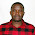This is a very powerful tutorial to use. Thanks

2.This is a very powerful tutorial to use. Thanks

1.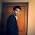3.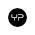Great explanation.
Thanks for sharing this.

1.Thank you for stopping by my blog. Cheers!

4.Thanks for the tips!

5.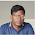thanks sir !

6.thanks sir !

7.wow...i feel my head somewhat heavy now...but its a very good example
Keep the good work up..thanks..!!

1.Glad you found it useful. Thanks!

8.excelente muchas gracias!!!!
no habrá algo de regresion logistica?

1.Check out this tutorial - http://www.listendata.com/2016/02/Logistic-Regression-with-R.html

9.Excelent explanation! Thanks.

1.10.All the picture link broken (please check the source of images) but very useful tutorial indeed

1.Are you seeing this tutorial from your workplace? Most of the companies block photo sharing websites.

11.Very cool and useful. Thanks

1.Thank you for stopping by my blog and sharing your feedback!

12.Very good job.
I think a partial residuals analysis is to be added in order to look at the type of relation between each independent variable and Y.

1.13.highlyCorrelated <- findCorrelation(descrCor, cutoff=0.6)

when i execute the above command its trowing error. the function not found. then i install the caret package after that also i am not able to get this function and execute this command, please help me. Thanks, in advance.

1.What's the error you are getting? Thanks!

2.> library(caret)
Error in loadNamespace(j <- i[[1L]], c(lib.loc, .libPaths()), versionCheck = vI[[j]]) :
there is no package called ‘pbkrtest’
package ‘caret’ was built under R version 3.2.5
Error: package or namespace load failed for ‘caret’

3.Even I'm getting the same error.
could you please tell me how it is resolved

14.Great work Deepanshu !!!
If can add a model that you have prepared and explain each point here nothing can beat that and using SAS ... Will be eagerly looking forward to it..
Thanks
Sumit

15.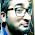Nice work Deepak !!

Can you please share the Linear Regression with SAS article also. Thanks.

16.Linear Regression with SAS is unfortunately missing. :-|
http://www.listendata.com/p/sas-tutorials.html

17.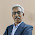Excellent Tutorial ....normally it's either Statistics or Programming ....here we find both well written!! Thanks a mill for sharing.

18.Great step by step tutorial in simple language !!! Do you have similar write ups in other algorithms Logit, random forests etc...

19.Good, Nice article

20.Simple, crisp and useful..Thank You :)

21.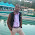this means now i solve one of my problems. thank u very much. would u mind if u give us the samme explanation on ANOVA(one way and two way)

22.Hi,
After I type below line of code:
fit = lm(mpg ~ ., data=dat3)

I am getting the error as : Error in lm(mpg ~ ., data = dat3) : unused argument (data = dat3)

1.23.Great post! Many thanks!

24.This comment has been removed by the author.

25.Is it the process we have to follow in realtime data analysis.?

26.There is no package called DAAG in my R studio.How i can install is it available only in R

27.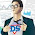good efforts

28.Is there any good dataset for practicing Linear Regression in R other than mtcars? I tried some datasets online but I found data is not good.

29.First of all thanks for providing this wonderful insight on "Linear Regression". I couldn't ask for better. I have a few questions (may be I am dumb). Hope you answer those :
In the beginning may I know why did you not consider MPG (according to the comments we infer that it is a highly dependent variable and so we are dropping that. But, how did we come to conclusion that it is a highly dependent variable? And again why are we considering it in building the LM model? Thanks in advance

1.It is because mpg is a dependent variable. In order to check multicollinearity which is high correlation between independent variables (not dependent variable). I think you got confused between dependent and independent variables.

30.I am start DS,

31.This is the best blog among all the ds blogs, Thanks for very fruitful information :)

I'm a newbie to data science, is this the standard procedure data scientists follow to solve the regression problem?

can i replicate the same method to all the problems and provide the inferences to clients.

32.wonderful sir...may you help me on the commands to transform data into long format I am very new to R programming.

33.Awesome blog. Keep up your good work. By the way I need some clarity on
1.Is there no other way than eliminating multicollinear variables?
2. Why don't we find their combined effect on dependent variable?

34.This is easy to understand and more powerful. The statistics concepts are totally new for me because I don't have maths & stat background even though I can understand this concepts easily with the help of this blog.

Good to learn....Thank you

35.Have you made any changes to the data? because i am getting these variables "cyl" "disp" "hp" "wt" "vs" "drat" as highly correlated at cutoff=0.7. If not, can you please tell me the reason why i am getting a different result?

1.I have not made any changes to the data. It's hard to say without looking your data. What's more important is that you understand the concepts and implementation in R.

36.Hi,

Right now I am on learning phase.I am having a question on why we are keeping cutoff ratio as 0.7?

Is there any limits? Help me to understand on this

37.Hi.

Thank you for the great tutorial.
I have two questions regarding the correlated variables:
1. Why did we drop all three highly correlated variables ("hp" "disp" "wt" )? Should we keep one of them?
2. Shouldn't we use absolute value cutoff for determine highly correlated variables?

38.Hi can you please post for Linear Regression in SAS, only for programming in SAS.
Thanks for the theory part its very clear.

39.Wonderful! Explanation!!

40.Hi, Deepanshu
First of all thanks for providing this wonderful insight on "Linear Regression".
I couldn't ask for better. I have a few questions (may be I am dumb). Hope you answer
when i execute the command

highlyCorrelated=findCorrelation(descrCor,cutoff=0.7)

its trowing error.

Error in findCorrelation(descrCor, cutoff = 0.7) :
could not find function "findCorrelation"

1.You need to install and load package named "caret" before using findCorrelation function.

2.Hi

I already installed caret package and tried.

Still getting same issue.

41.Hi just want to know during linear regression function you have deleted three features based on collinearity, but after doing forward and backward steps to find out useful features you did not include any of the removed 3 features may I know why?

1.You can try experimenting in including them and check collinearity again.

42.Error in findCorrelation(descrCor, cutoff = 0.7) :
could not find function "findCorrelation"

43.Error in findCorrelation(descrCor, cutoff = 0.7) :
could not find function "findCorrelation"

I installed all packages correctly
44.